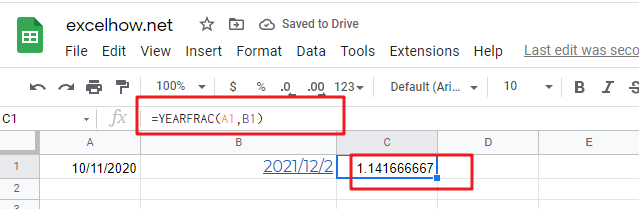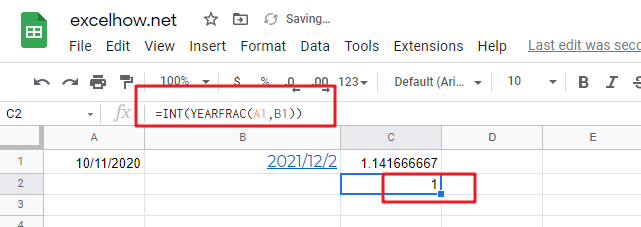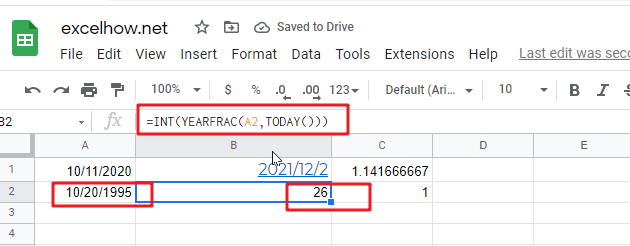# ExcelHow

This post will guide you how to use Google Sheets YEARFRAC function with syntax and examples.

## Description

The Google Sheets YEARFRAC function returns the number of years between two dates including fractional years. And you can use this function to calculate age with a birthdate.

The YEARFRAC function can be used to get a decimal value that represents fractional years between two given dates in google sheets. The purpose of this function is to get the fraction of a year between two dates and its returned value is a decimal number.

The YEARFRAC function is a build-in function in Google Sheets and it is categorized as a Date function.

## Syntax

The syntax of the YEARFRAC function is as below:

=YEARFRAC(start_date, end_date, [day_count_convention])

Where the YEARFRAC function arguments are:

• Start_dateThis is a required argument. The starting date from which you want to count the number of years. It must be a cell reference that containing a date, or a function that returning a date type or a number.
• End_date – This is a required argument. The end date that is considering in the calculation. It must be a cell reference that containing a date, or a function that returning a date type or a number.
• day_count_convention This is an optional argument.an type of what day count method to use.
 type calculation 0(default) 30/360 1 Actual/actual 2 Actual/360 3 Actual/365 4 30/360

Note:

• this function is mostly used in a financial setting.
• You need to make sure that the inputs to the function are either cell reference that containing valid dates.

## Google Sheets YEARFRAC Function Examples

The below examples will show you how to use google sheets YEARFRAC Function to return the number of years between two dates.

1# a start date is in Cell A1, and an end date is in Cell B1, and using the YEARFRAC function to count the years between two dates:

`=YEARFRAC(A1,B1)`2# if you want to gt a whole number , and you can use the INT function in combination with the YEARFRAC function, type:

`=INT(YEARFRAC(A1,B1)`3# if you want to get the current age based on a birthdate in Cell A2, and you can use the following formula based on the INT function, the TODAY function and the YEARFRAC function, type:

`=INT(YEARFRAC(A2,TODAY()))`